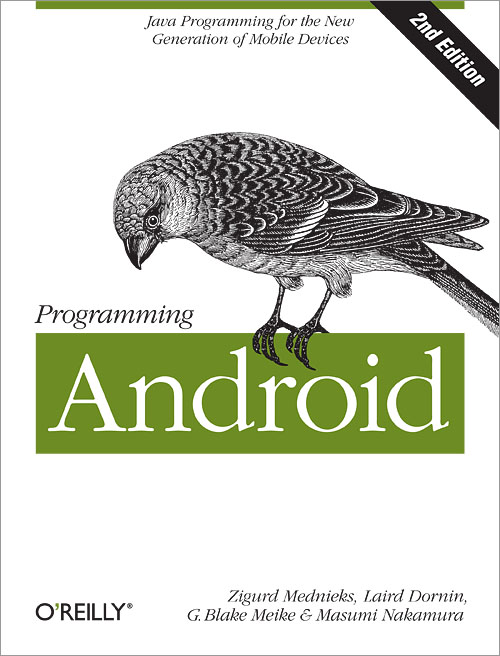## Thursday, February 21, 2019

### Computer Fundamentals (Objective Questions With Answers)

File Size: 170 Kb

1. Which of the following involves digital Quantities?
A. Ten Position switch
B. Current Meter
C. Temperature

2. Which of the following choices is NOT a Characteristic of analog Quantity?
A. Variable amplitude
B. One quantity is represented by another which is proportional to the First
C. Is considered discrete
D. They can very over a continuous range of values

3. The decimal system is composed of ______ numerical or symbols.
A. 2
B. 10
C. 8
D. 16

4. Change in state is
A. same state
B. reset
C. set
D. Toggle

5. What is the decimal equivalent of (1101011)2?
A. 107
B. 108
C. 96
D. 100

6. What is the next binary number following (10111)2 in the counting
A. 11100
B. 11001
C. 10110
D. 11000

7. What is the largest decimal value that can be represented using 12 bits?
A. 144
B. 2048
C. 4095
D. 4096

8. What is the largest number that can be represented using 8 bits?
A. 11111111
B. 10111011
C. 10111111
D. 11011111

9. A digital circuit is also referred to as a/an _______ circuit.
A. arithmetic
B. logic
C. electrical
D. sequential

10. CMOS means
A. Complementary Main-Oxide Semiconductor
B. Complementary Metal-Oxide Semiconductor
C. Complements Main-Oxidation Semiconductor
D. Correlation in Metal Oxidized SemiconductorProgramming Android